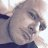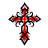# Is This a Repainting Indicator?

D

#### David45

##### Member
2019 Donor
Hi All,

Can someone please confirm if this is a repainting indicator or do the arrows appear on the actual day of the trend reversal? Many thanks!

# Trend Reversal Scanner
# Dixon72 with modifications by tomsk
# 1.18.2020
# Scanner by https://usethinkscript.com/u/theelderwand

# V1.0 - 11.09.2019 - Dixon72 - Initial release of Trend Reversal Scanner
# V1.1 - 01.18.2020 - tomsk - Modified to only display last bullish/bearish signal

def price = close;
def superfast_length = 9;
def fast_length = 14;
def slow_length = 21;
def displace = 0;

def mov_avg9 = ExpAverage(price[-displace], superfast_length);
def mov_avg14 = ExpAverage(price[-displace], fast_length);
def mov_avg21 = ExpAverage(price[-displace], slow_length);

#moving averages
def Superfast = mov_avg9;
def Fast = mov_avg14;
def Slow = mov_avg21;

def buy = mov_avg9 > mov_avg14 and mov_avg14 > mov_avg21 and low > mov_avg9;
def stopbuy = mov_avg9 <= mov_avg14;

def sell = mov_avg9 < mov_avg14 and mov_avg14 < mov_avg21 and high < mov_avg9;
def stopsell = mov_avg9 >= mov_avg14;
def sellnow = !sell and sell;
def sellsignal = CompoundValue(1, if sellnow and !stopsell then 1 else if sellsignal == 1 and stopsell then 0 else sellsignal, 0);
def Sell_Signal = sellsignal == 0 and sellsignal;

input method = {default average, high_low};
def bubbleoffset = .0005;
def percentamount = .01;
def revAmount = .05;
def atrreversal = 2.0;
def atrlength = 5;
def pricehigh = high;
def pricelow = low;
def averagelength = 5;
def averagetype = AverageType.EXPONENTIAL;
def mah = MovingAverage(averagetype, pricehigh, averagelength);
def mal = MovingAverage(averagetype, pricelow, averagelength);
def priceh = if method == method.high_low then pricehigh else mah;
def pricel = if method == method.high_low then pricelow else mal;
def EI = ZigZagHighLow("price h" = priceh, "price l" = pricel, "percentage reversal" = percentamount, "absolute reversal" = revAmount, "atr length" = atrlength, "atr reversal" = atrreversal);
rec EISave = if !IsNaN(EI) then EI else GetValue(EISave, 1);
def chg = (if EISave == priceh then priceh else pricel) - GetValue(EISave, 1);
def isUp = chg >= 0;
def EIL = if !IsNaN(EI) and !isUp then pricel else GetValue(EIL, 1);
def EIH = if !IsNaN(EI) and isUp then priceh else GetValue(EIH, 1);
def dir = CompoundValue(1, if EIL != EIL or pricel == EIL and pricel == EISave then 1 else if EIH != EIH or priceh == EIH and priceh == EISave then -1 else dir, 0);
def signal = CompoundValue(1, if dir > 0 and pricel > EIL then if signal <= 0 then 1 else signal else if dir < 0 and priceh < EIH then if signal >= 0 then -1 else signal else signal, 0);

def bullish2 = if signal > 0 and signal <= 0 then barNumber() else 0;
plot upArrow = if barNumber() == HighestAll(bullish2) then low * 0.994 else Double.NaN;
upArrow.SetPaintingStrategy(PaintingStrategy.BOOLEAN_ARROW_UP);
upArrow.SetLineWeight(5);
upArrow.SetDefaultColor(Color.CYAN);

def bearish2 = if signal < 0 and signal >= 0 then barNumber() else 0;
plot downArrow = if barNumber() == HighestAll(bearish2) then high * 1.004 else Double.NaN;
downArrow.SetPaintingStrategy(PaintingStrategy.BOOLEAN_ARROW_DOWN);
downArrow.SetLineWeight(5);
downArrow.SetDefaultColor(Color.YELLOW);Can this be used to make a repainting study no longer repaint in TOS? Questions 2Xtreme Vortex Bundle Indicator for ThinkorSwim Indicators 13